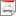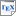[YKNP04] H. Younes, M. Kwiatkowska, G. Norman and D. Parker. Numerical vs. Statistical Probabilistic Model Checking: An Empirical Study. In K. Jensen and A. Podelski (editors), Proc. TACAS'04, volume 2988 of Lecture Notes in Computer Science, pages 46-60, Springer. March 2004. [ps.gz] [pdf] [bib] Downloads:ps.gz (143 KB)pdf (307 KB)bib Notes: The original publication is available at link.springer.com. Links: [Google] [Google Scholar] [CiteSeer] Abstract. Numerical analysis based on uniformisation and statistical techniques based on sampling and simulation are two distinct approaches for transient analysis of stochastic systems. We compare the two solution techniques when applied to the verification of time-bounded until formulae in the temporal stochastic logic CSL. This study differs from most previous comparisons of numerical and statistical approaches in that CSL model checking is a hypothesis testing problem rather than a parameter estimation problem. We can therefore rely on highly efficient sequential acceptance sampling tests, which enables statistical solution techniques to quickly return a result with some uncertainty. This suggests that statistical techniques can be useful as a first resort during system prototyping, rather than as a last resort as often suggested. We also propose a novel combination of the two solution techniques for verifying CSL queries with nested probabilistic operators.